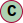# Class RelativeLinearVelocity

Relative linear velocity to another reference object

#### Hierarchy-Diagram##### Legendclass

## Properties

referenceObjectType: string
referenceObjectUID: string

Reference object UID that this location is relative to

referenceValue: LinearVelocity
timestamp: number = ...

Position recording timestamp

## Accessors

• get accuracy(): Accuracy<U, any>
• Position accuracy

### Returns

Position accuracy

#### Returns Accuracy<U, any>

• set accuracy(value: Accuracy<U, any>): void
• Position accuracy

#### Returns void

• get probability(): number
• Get the position probability

### Returns

Probability between 0 and 1

#### Returns number

• set probability(value: number): void
• Position probability

#### Returns void

• get velocity(): LinearVelocity
• #### Returns LinearVelocity

• set velocity(value: LinearVelocity): void